Quick Notes Electron Orbitals

• Electrons are negatively charged, so they are attracted to the positive charge of a nucleus (electrostatic attraction).
• The closer an electron is to a nucleus, the lower its energy.
• All electrons in an atom want to get as close to the nucleus as possible, but they repel each other.
• This forces electrons to exist in set regions called orbitals.
• One orbital can hold two electrons (one pair).
• Different orbitals have different shapes and energies. Orbital types are labelled with letters – s, p, d and f.
• Shells describe how far away an electron is from a nucleus, and therefore its energy.
• Shell 1 is the closest shell to the nucleus, shell 2 the next closest, etc.
• Due to different shapes, orbitals within a shell can have different energies and form sub-shells.
• Shells and sub-shells are filled successively – lowest available energy sub-shells and orbitals are filled first.

Full Notes Electron Orbitals

Electrons are negatively charged, meaning they are attracted to the positive charge of a nucleus and repel each other. Getting closer to a nucleus lowers the energy of an electron, but repulsion between electrons increases their energy.

As two oppositely charged particles get closer together, their energies lower. This means electrons closer to the positively charged nucleus of an atom are at a lower energy than electrons further away from the nucleus.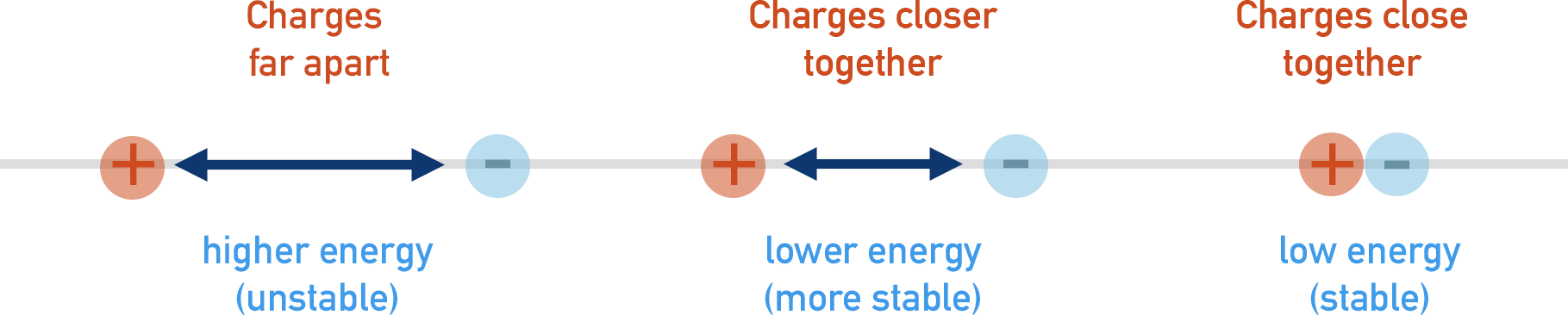To minimise repulsion, electrons do not simply cram around a nucleus, but arrange themselves in specific ways to keep their energies as low as possible.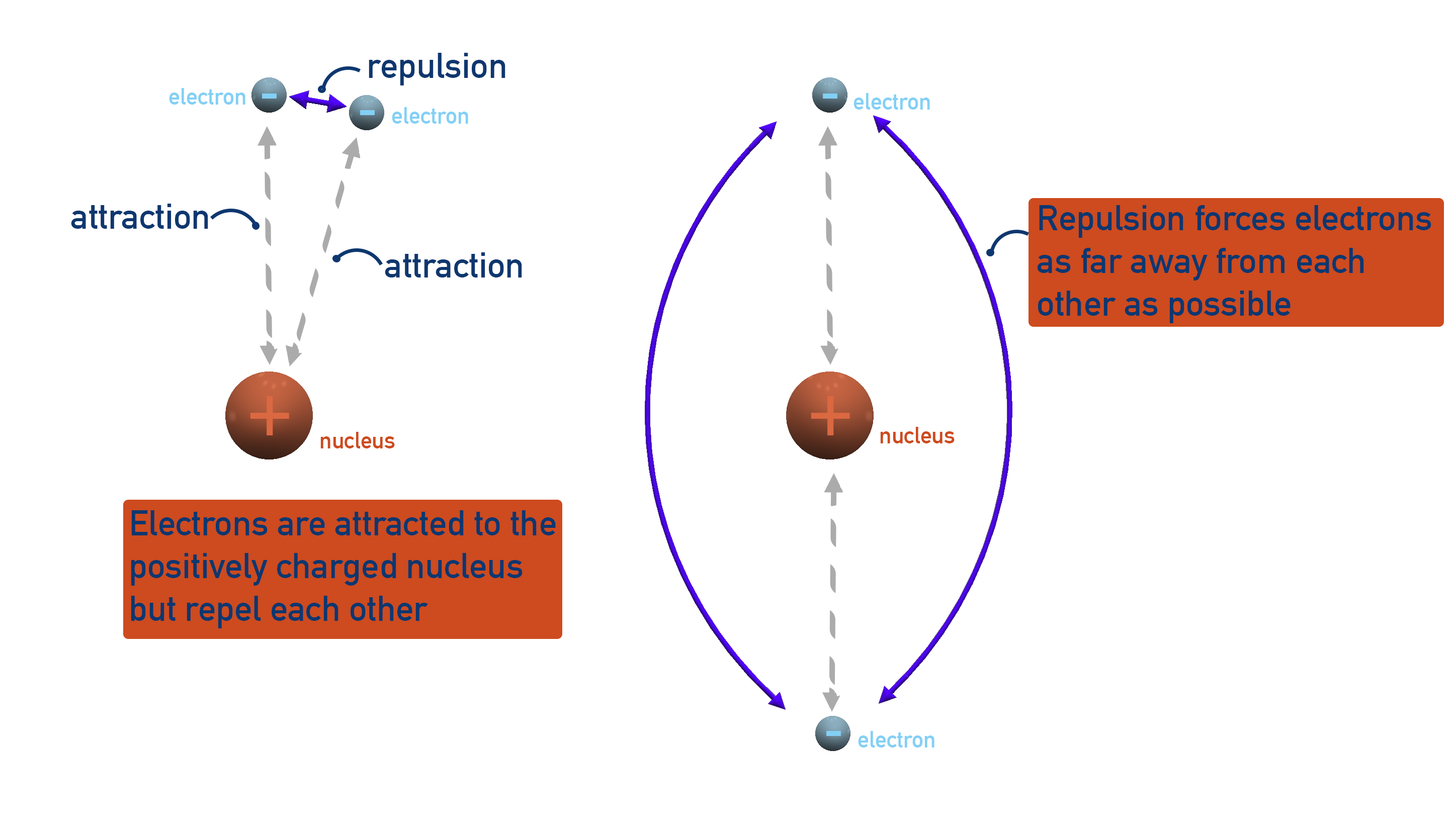Such specific arrangements of electrons are called orbitals. One orbital can hold two electrons (one pair). There are different shapes of orbitals to keep electron repulsion to a minimum. The orbital shapes studied at A-level are s, p and d (see below).

Very simply – an orbital is just the space around a nucleus that a pair of electrons is ‘allowed’ to be in.

There are only so many ways that electrons can arrange themselves to maximise their attraction to the nucleus but minimise their repulsion to each other. This means that some electrons have to move further away from the nucleus than others.

When all orbitals at a particular distance from the nucleus are full, any more electrons must fill orbitals further away from the nucleus. We say that an electron then enters a new shell.

The further a shell is from the nucleus, the higher its energy.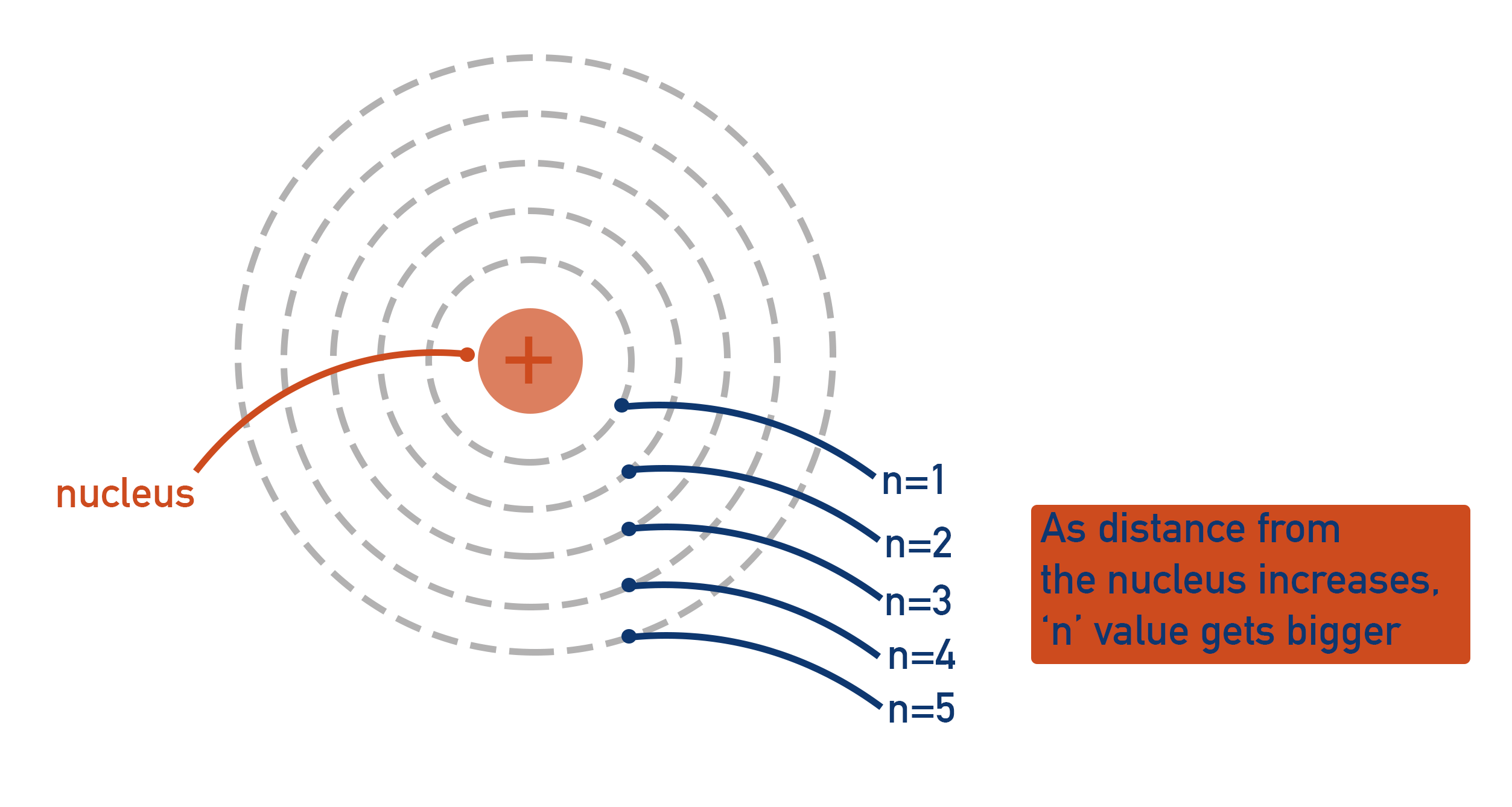A shell just indicates how far an electron is from the nucleus of an atom and the relative energy that is has. It is completely pointless measuring this distance with a unit! Instead, we use basic integers (whole numbers). The closest shell to the nucleus is assigned the number one (n = 1), the second closest shell is assigned the number two (n = 2), the third closest shell the number three (n = 3) etc.

The shells are always filled in order. Electrons will fill the first shell before the second and the second before the third. Think of a multi-story car park – lower levels fill first as the people in the cars want to be as close to the ground as possible. But, if the lower levels are already taken, they must park at a higher level.

Within each shell, different shaped orbitals have different energies. These energy levels are referred to as ‘sub-shells’ and explain the unique behaviour of many elements.

Orbital Shapes

Orbitals can have different shapes and some are larger than others. This means some orbitals have to be further away from the nucleus.

An s-orbital is a simple sphere that is close to the nucleus. Therefore, it is easy to fit in the first shell.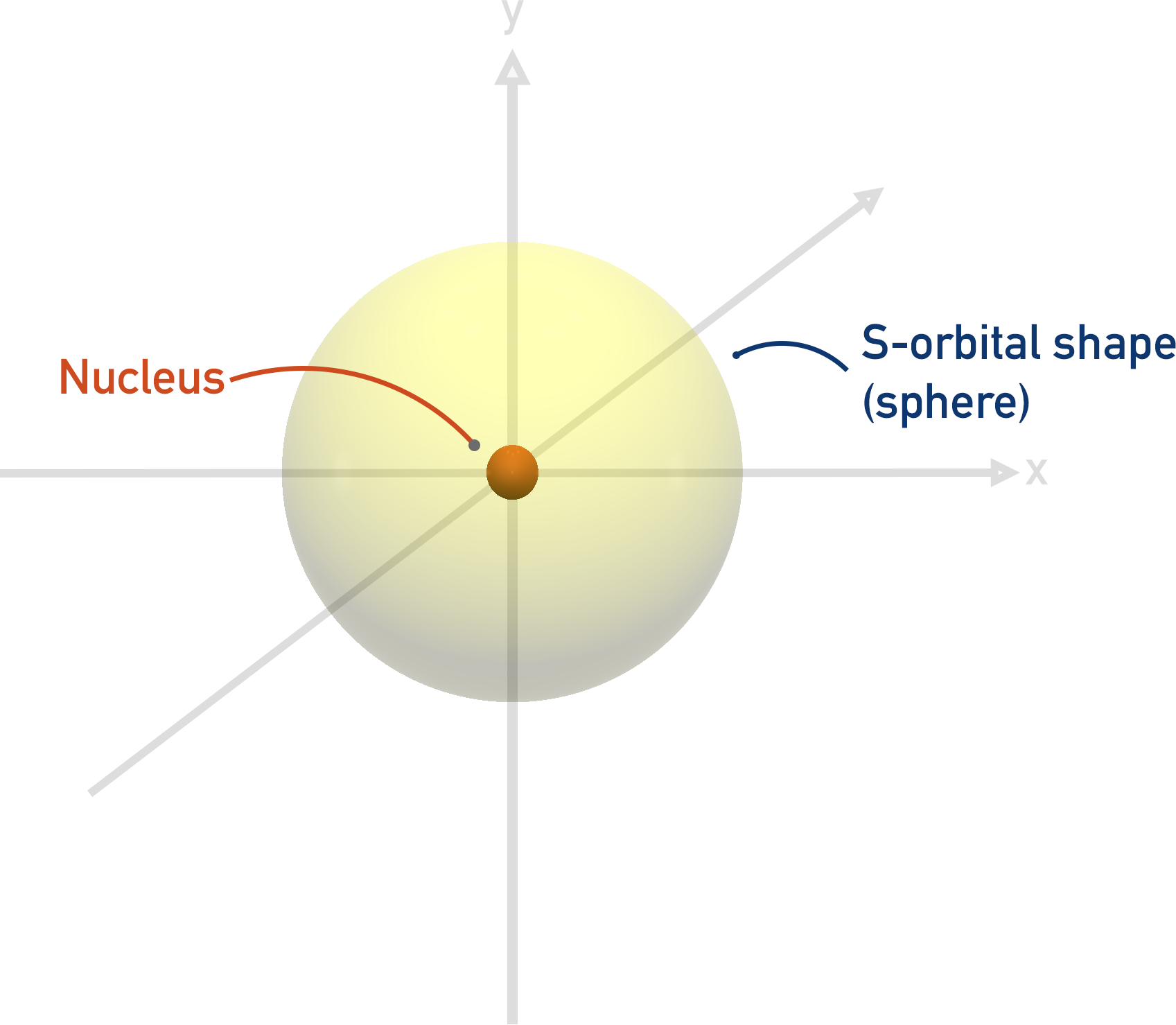A p-orbital is more like a dumb-bell shape, and extends out further than an s-orbital (this explains why the p-orbital is a higher energy orbital than the s-orbital). It is not possible to fit a p-orbital shape in the first shell (n=1).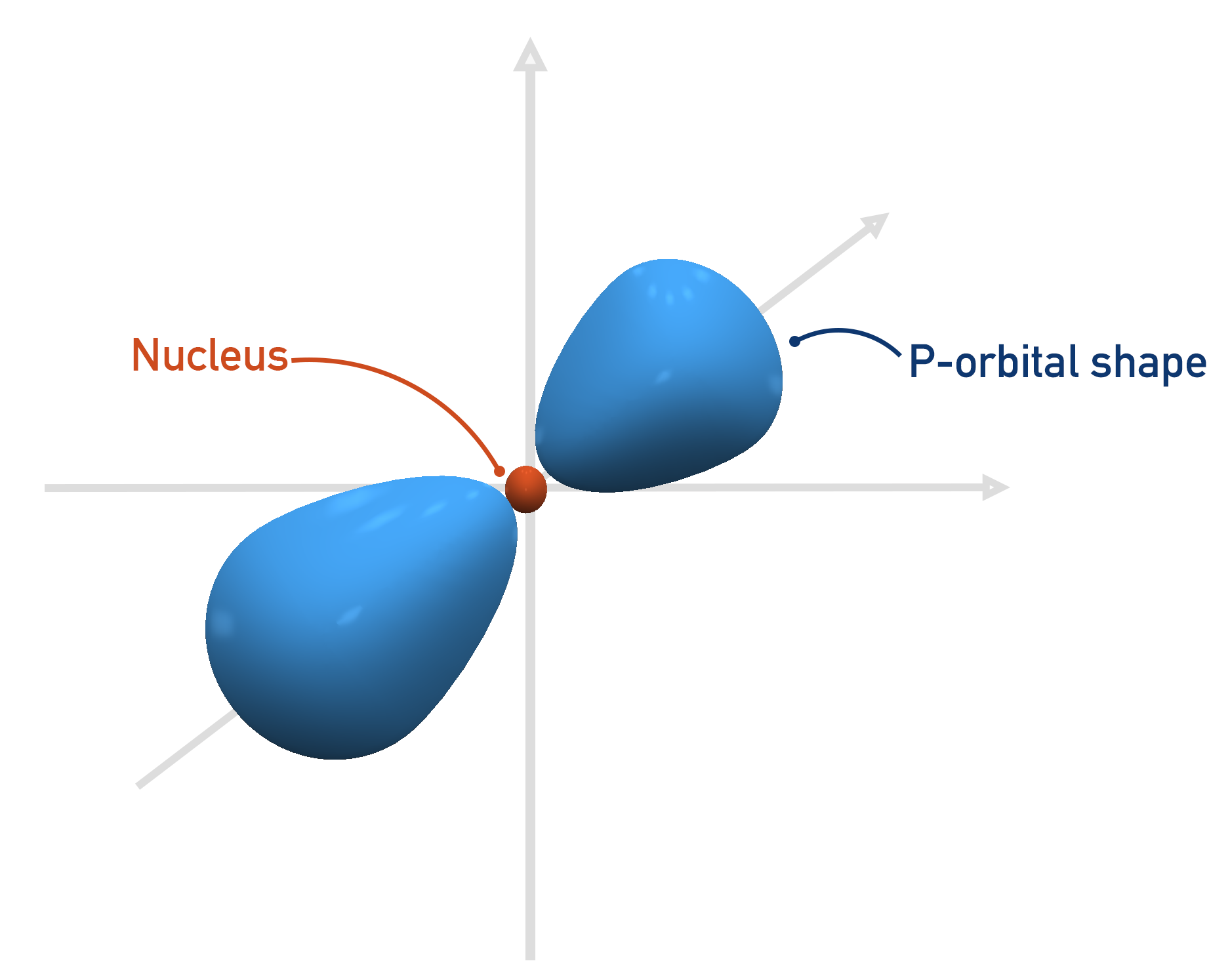The first shell that can have a p-orbital is the second, n = 2. There are three p-orbitals and they all have the same energies when full, they just ‘point’ in different directions.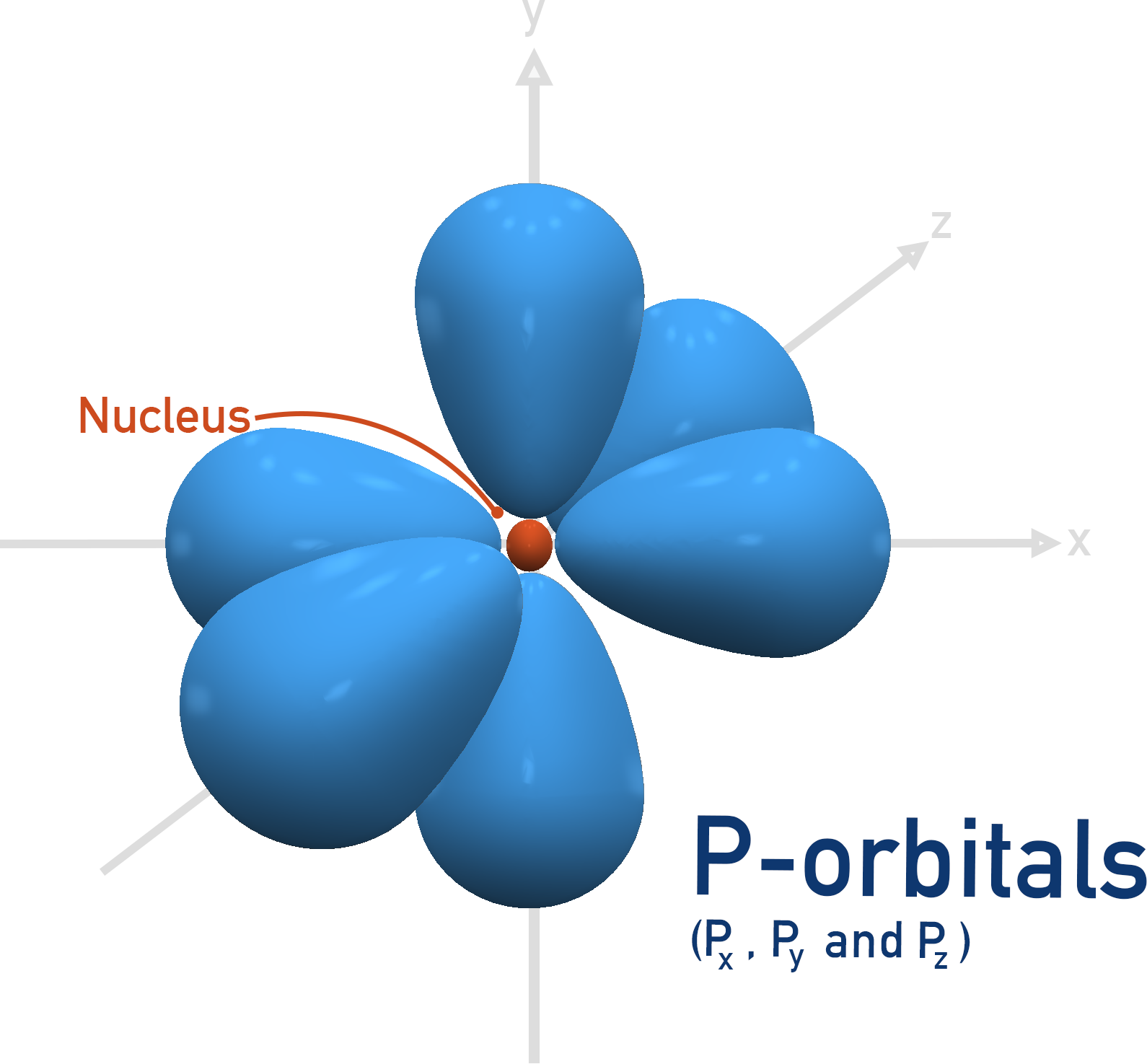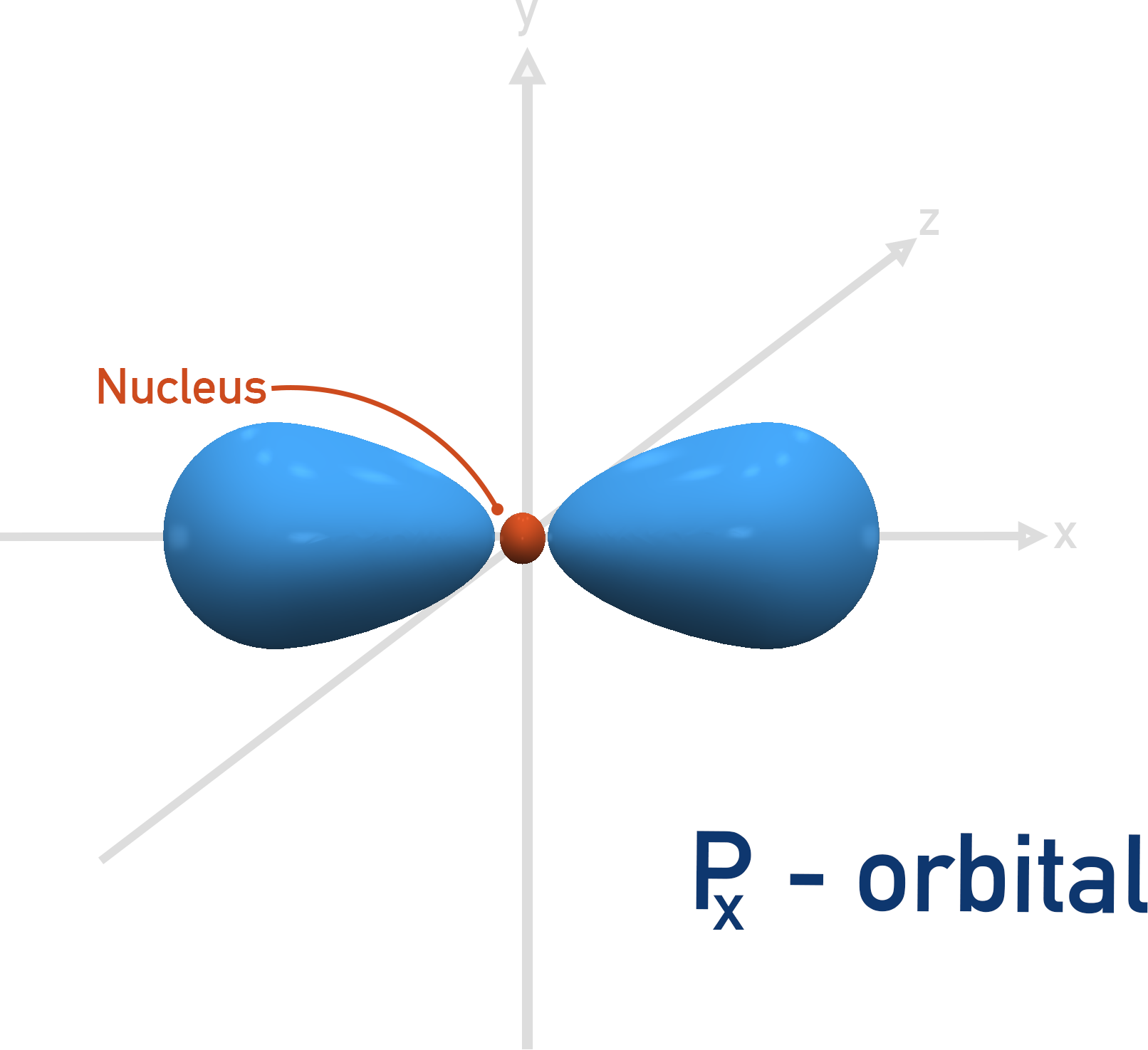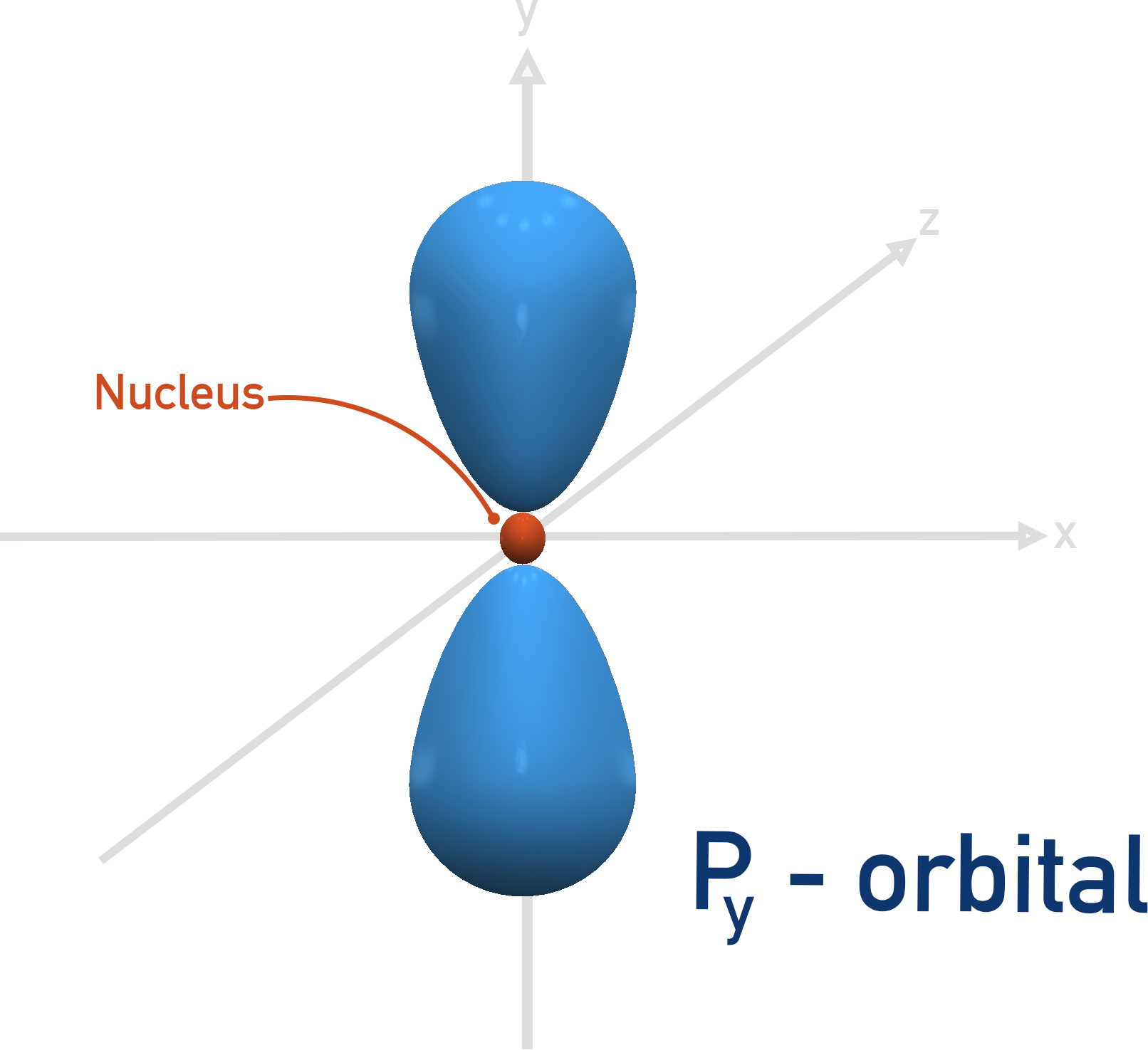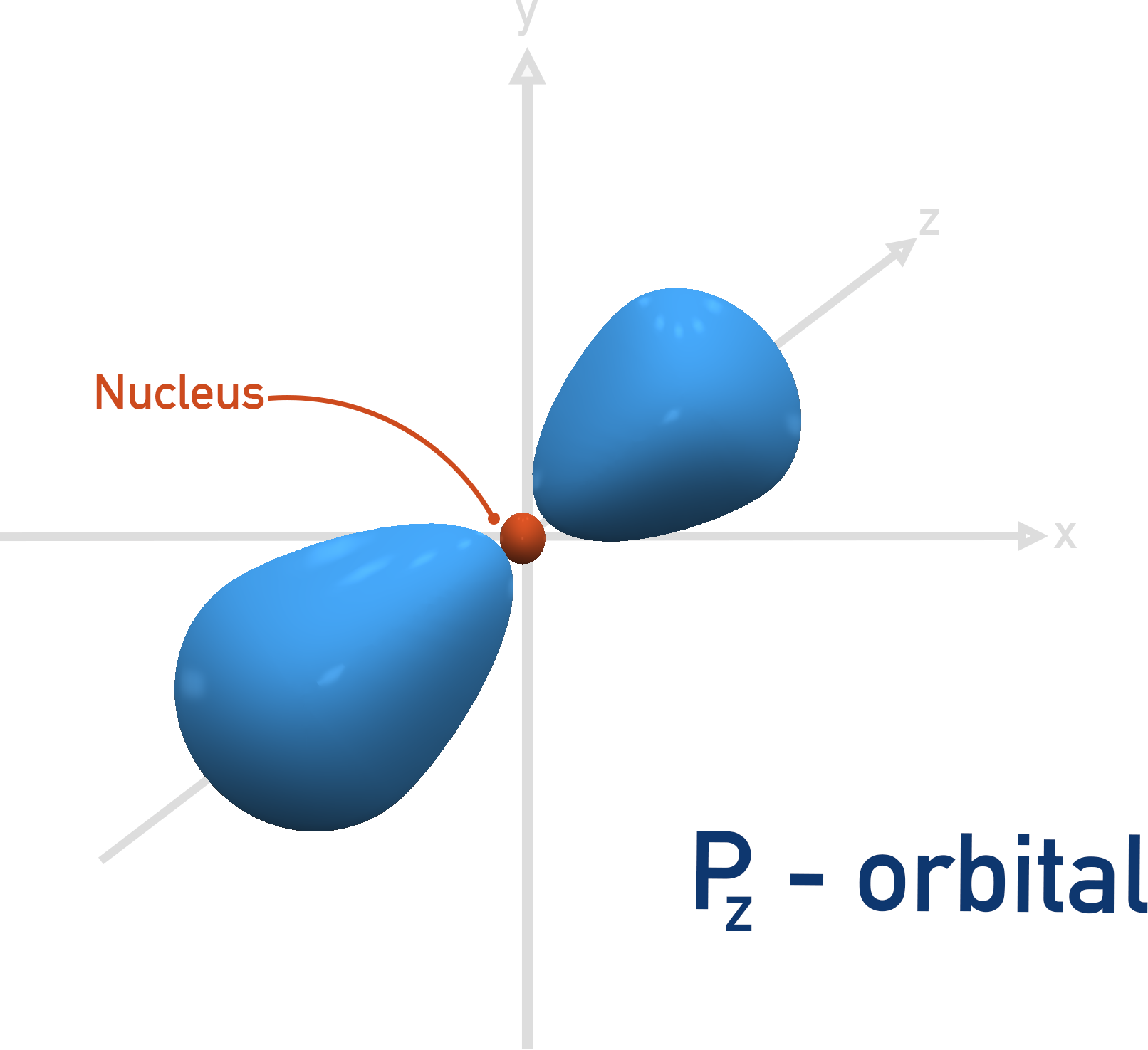D-orbitals have complicated shapes that occupy more space overall than the p-orbitals. In the same way a p-orbital can’t fit in the first shell, d-orbitals cannot fit in the second shell. This means there are no d-orbitals until the third shell, n = 3.

As electrons go into a new shell, the shape of the orbitals (s, p and d) are the same as for lower energy shells, they are just now larger.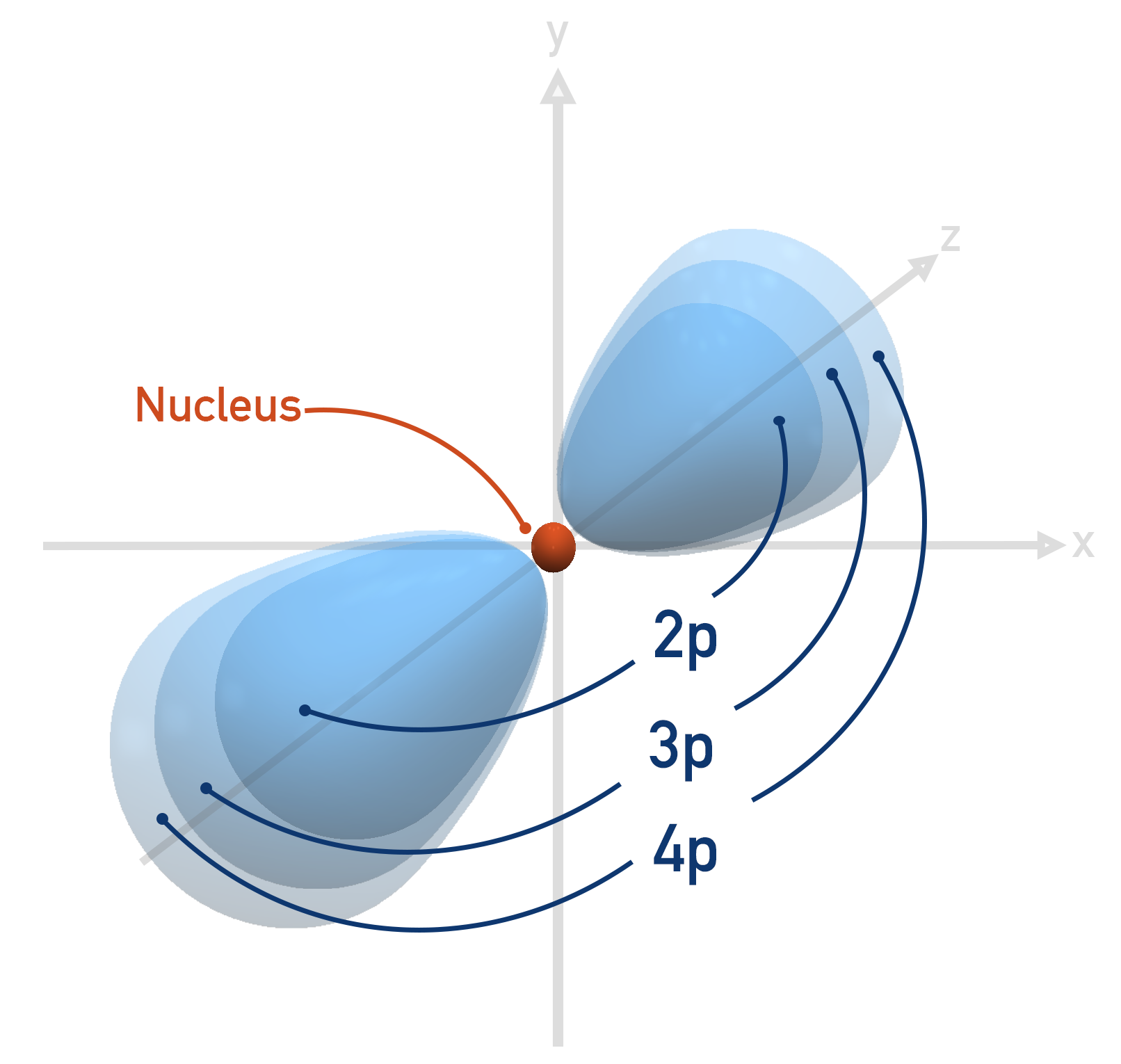Do electron orbitals actually look like the images shown?

In truth, no.

A bit extra: electrons move very fast continually, so we cannot ever say exactly where an electron will be at any one time. As soon as an electron is in one place, it moves to another. These orbital diagrams and shapes are probability densities. They show the area where an electron is most likely to be found at a given time. It is a bit like saying someone is at home. You can say that the person is within their house, but you have no real way to know where the person is exactly – all you can say is ‘you should find them somewhere in their house’. The orbital diagrams are basically the electrons' houses – they can be anywhere in the given areas.

Notes:

This page covers electron orbitals in the level of detail applicable to A-level Chemistry. The exact shapes, energies and behaviour of electron orbitals are determined by a branch of physics and chemistry called quantum mechanics, where electrons are treated as waves and particles. By treating electrons as waves, wave functions for each electron within an atom can be used to determine where the electron is likely to be found, producing ‘probability densities’. It is these probability densities that are used to produce the simplified ‘orbital diagrams’ and shapes that are often used (as on this page) to represent electron orbitals.